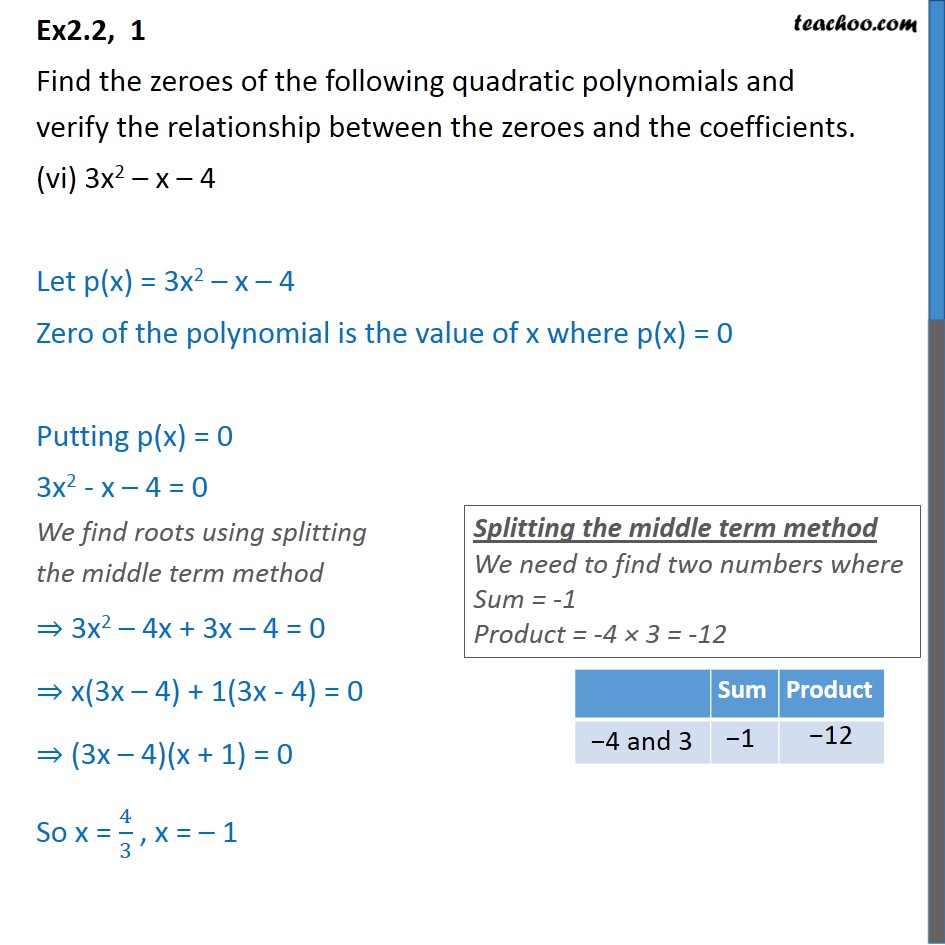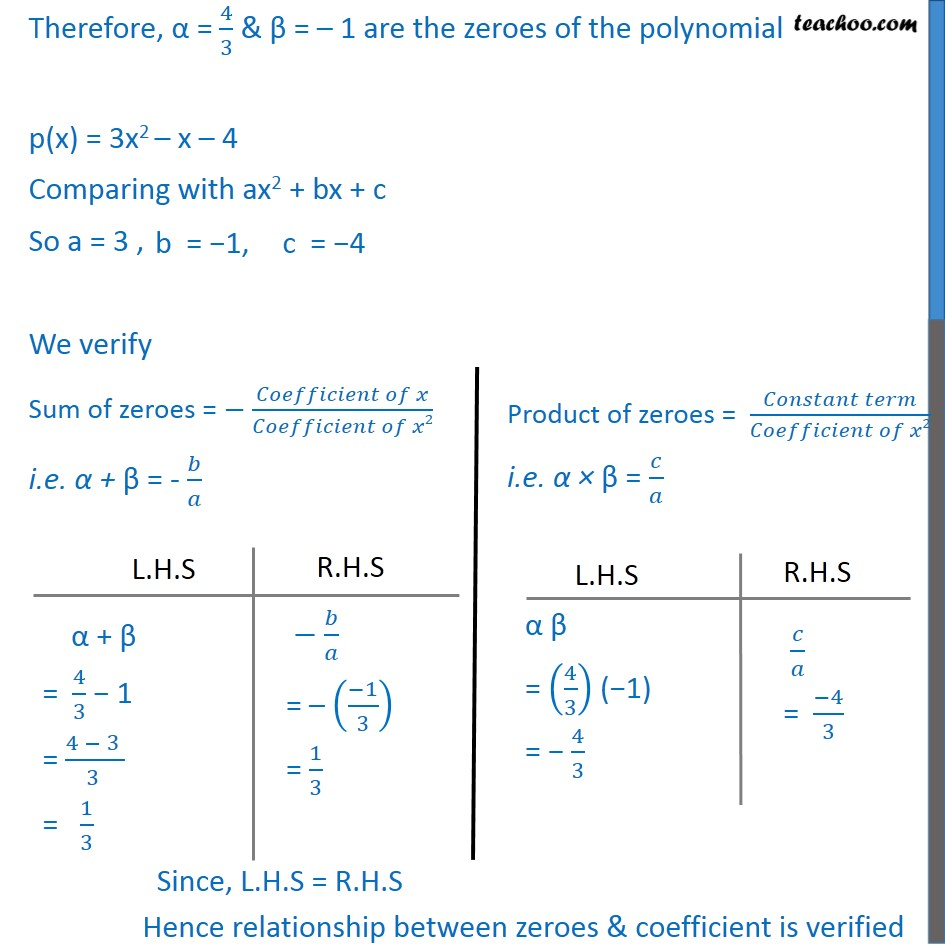1. Chapter 2 Class 10 Polynomials (Term 1)
2. Serial order wise
3. Ex 2.2

Transcript

Ex2.2, 1 Find the zeroes of the following quadratic polynomials and verify the relationship between the zeroes and the coefficients. (vi) 3x2 – x – 4 Let p(x) = 3x2 – x – 4 Zero of the polynomial is the value of x where p(x) = 0 Putting p(x) = 0 3x2 - x – 4 = 0 We find roots using splitting the middle term method ⇒ 3x2 – 4x + 3x – 4 = 0 ⇒ x(3x – 4) + 1(3x - 4) = 0 ⇒ (3x – 4)(x + 1) = 0 So x = 4/3 , x = – 1 Therefore, α = 4/3 & β = – 1 are the zeroes of the polynomial p(x) = 3x2 – x – 4 Comparing with ax2 + bx + c So a = 3 , We verify Sum of zeroes = − (𝐶𝑜𝑒𝑓𝑓𝑖𝑐𝑖𝑒𝑛𝑡 𝑜𝑓 𝑥)/(𝐶𝑜𝑒𝑓𝑓𝑖𝑐𝑖𝑒𝑛𝑡 𝑜𝑓 𝑥2) i.e. α + β = - 𝑏/𝑎